# Function Overloading in C++ - Learn C++ - C++ Tutorial - C++ programming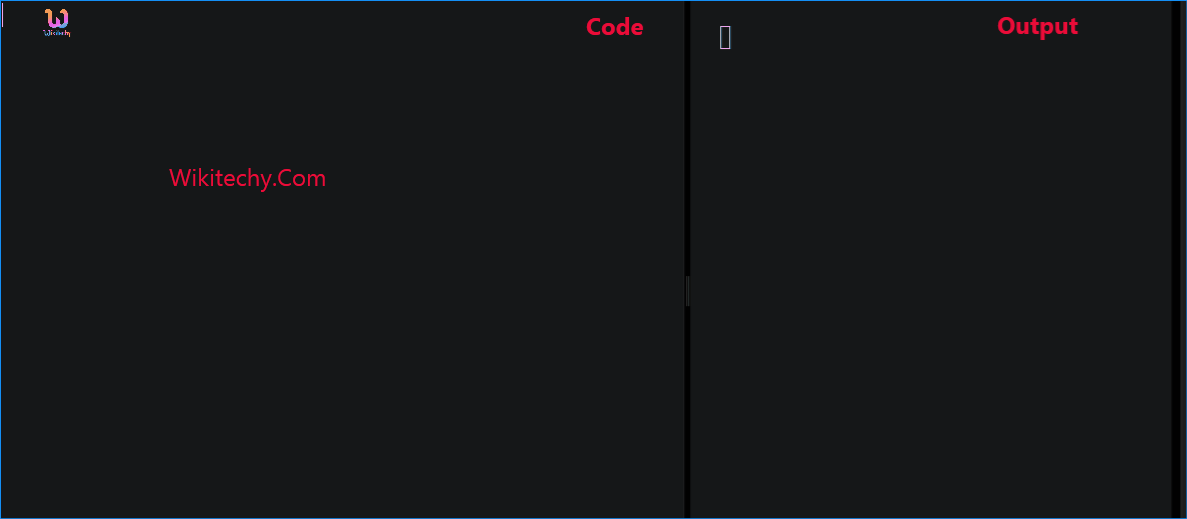Learn c++ - c++ tutorial - function overloading in c++ - c++ examples - c++ programs

## Functions in C++:

• Functions are used to provide modularity to a program. A function is a block of code that performs some operation.
•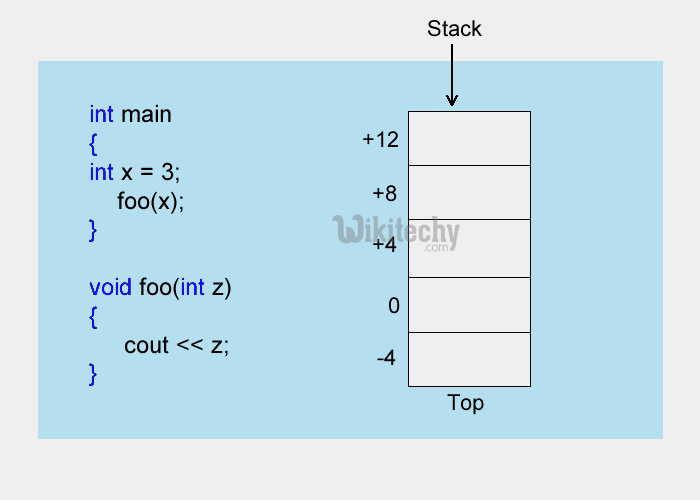learn c++ tutorials - functions in c++ Example

• A function can optionally define input parameters that enable callers to pass arguments into the function.
• A function can optionally return a value as output.

## Syntax of Function:

• return-type : suggests what the function will return. It can be int, char, some pointer or even a class object. There can be functions which does not return anything, they are mentioned with void.
• Function Name : is the name of the function, using the function name it is called.
• Parameters : are variables to hold values of arguments passed while function is called. A function may or may not contain parameter list.
• Here, a and b are sent as arguments, and x and y are parameters which will hold values of a and b to perform required operation inside function.
• Depending on whether a function is predefined or created by programmer; there are two types of function:
1. Library Function
2. User-defined Function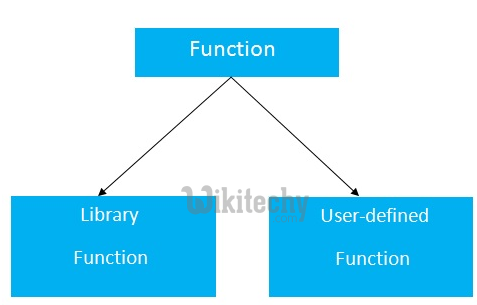## Library Function:

• Library functions are the built-in function in C++ programming.
• Programmer can use library function by invoking function directly; they don't need to write it themselves.

## Output:

• In the example above, sqrt() library function is invoked to calculate the square root of a number.
• Notice code #include in the above program. Here, cmath is a header file. The function definition of sqrt()(body of that function) is present in the cmath header file.
• You can use all functions defined in cmath when you include the content of file cmath in this program using #include .
• Every valid C++ program has at least one function, that is, main() function.

## User-defined Function:

• C++ allows programmer to define their own function.
• A user-defined function groups code to perform a specific task and that group of code is given a name(identifier).
• When the function is invoked from any part of program, it all executes the codes defined in the body of function.

## Example 1: User Defined Function

• C++ program to add two integers. Make a function add() to add integers and display sum in main() function.

## Function prototype (declaration)

• If a user-defined function is defined after main() function, compiler will show error. It is because compiler is unaware of user-defined function, types of argument passed to function and return type.
• In C++, function prototype is a declaration of function without its body to give compiler information about user-defined function.
• Function prototype in the above example is:
• You can see that, there is no body of function in prototype. Also, there are only return type of arguments but no arguments. You can also declare function prototype as below but it's not necessary to write arguments.
• Note: It is not necessary to define prototype if user-defined function exists before main()function.

## Function Call:

• To execute the codes of function body, the user-defined function needs to be invoked(called).
• In the above program, add(num1,num2); inside main() function calls the user-defined function.
•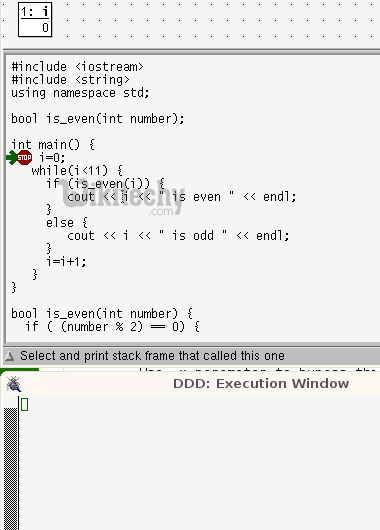learn c++ tutorials - function in c++ Example

• The function returns an integer which is stored in variable add.

## Function Definition:

• The function itself is referred as function definition. Function definition in the above program is:
• When the function is called, control is transferred to the first statement of the function body.
• Then, other statements in function body are executed sequentially.
• When all codes inside function definition is executed, control of program moves to the calling program.

## Passing Arguments to Function:

• In programming, argument (parameter) refers to the data which is passed to a function (function definition) while calling it.
• In the above example, two variables, num1 and num2 are passed to function during function call. These arguments are known as actual arguments.
• The value of num1 and num2 are initialized to variables a and b respectively. These arguments a and b are called formal arguments.
• This is demonstrated in figure below: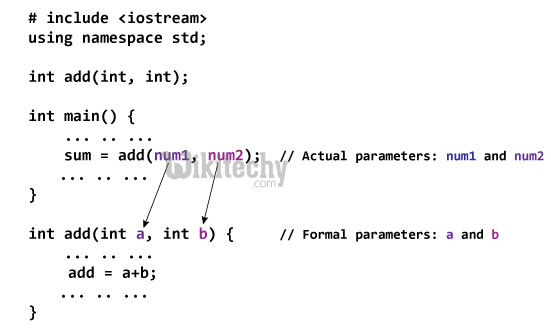## Notes on passing arguments:

• The numbers of actual arguments and formals argument should be the same. (Exception: Function Overloading)
• The type of first actual argument should match the type of first formal argument. Similarly, type of second actual argument should match the type of second formal argument and so on.
• You may call function a without passing any argument. The number(s) of argument passed to a function depends on how programmer want to solve the problem.
• You may assign default values to the argument. These arguments are known as default arguments.
• In the above program, both arguments are of int type. But it's not necessary to have both arguments of same type.